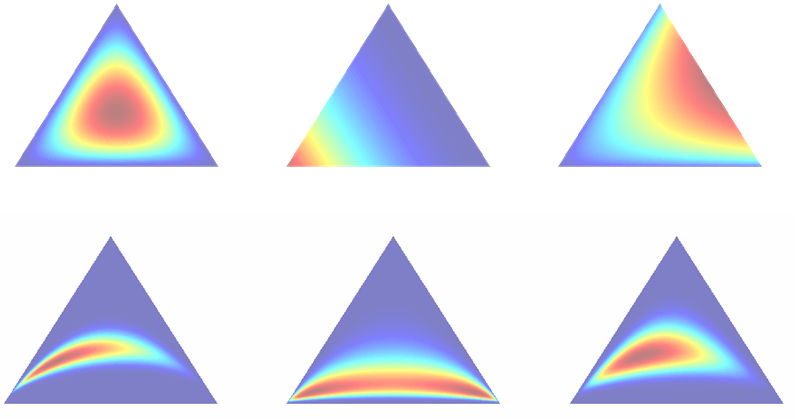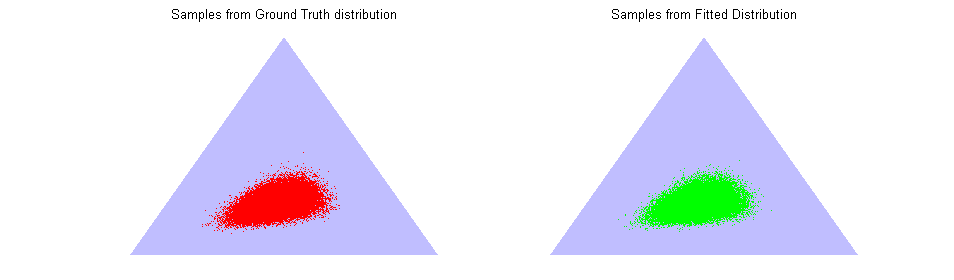# HLNFit

### Matlab code for fitting a Hierarchical Logistic-Normal Distribution

by Jonathan Huang and Tomasz Malisiewicz

Are Dirichlet Distributions too simple for you?
Are you getting tired of the Exponential Family?

... Then the Logistic Normal Distribution might be right up your alley!Top Row: Dirichlet Distributions, Bottom Row: Logistic-Normal Distributions

The generative process for the Hierarchical Logistic-Normal Distribution can be summarized as follows:
1. Draw V from a Multivariate Gaussian, N(μ,Σ).
2. Exponentiate V.
3. Project to the Simplex to get a probability vector P (At this point we have a Logistic-Normal distribution).
4. Draw N samples from Multinomial(P).

Algorithm Details (pdf)

List of included .m Files
• hlnscript.m - example script which illustrates code usage
• hlnEM.m - EM scheme for fitting an HLN distribution
• conditionalMode.m - hlnEM subroutine
• plotlogisticnormal.m - Plot samples from a Logistic Normal on a 2-Simplex
• plotLNlevelsets.m - Plot level sets of a Logistic Normal pdf on a 2-Simplex
• minimize.m - Carl Rasmussen's Conjugate Gradient code
• genHLNsamples.m - Generate samples from a Hierarchical Logistic-Normal Distribution
• genSamps.m - Generate samples from a Logistic-Normal distribution

Instructions:
1. First make sure you have the Matlab Statistics Toolbox.
2. Unzip hlnfit.zip.
3. Open Matlab and run hlnscript
The output of hlnscript should look something like the following figure.Click to go Back